# Ford-Fulkerson Algorithm

In this tutorial, you will learn what Ford-Fulkerson algorithm is. Also, you will find working examples of finding maximum flow in a flow network in C, C++, Java and Python.

Ford-Fulkerson algorithm is a greedy approach for calculating the maximum possible flow in a network or a graph.

A term, flow network, is used to describe a network of vertices and edges with a source (S) and a sink (T). Each vertex, except S and T, can receive and send an equal amount of stuff through it. S can only send and T can only receive stuff.

We can visualize the understanding of the algorithm using a flow of liquid inside a network of pipes of different capacities. Each pipe has a certain capacity of liquid it can transfer at an instance. For this algorithm, we are going to find how much liquid can be flowed from the source to the sink at an instance using the network.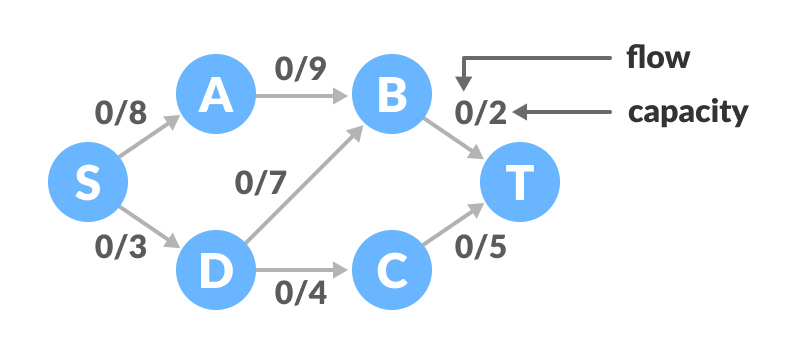Flow network graph

## Terminologies Used

### Augmenting Path

It is the path available in a flow network.

### Residual Graph

It represents the flow network that has additional possible flow.

### Residual Capacity

It is the capacity of the edge after subtracting the flow from the maximum capacity.

## How Ford-Fulkerson Algorithm works?

The algorithm follows:

1. Initialize the flow in all the edges to 0.
2. While there is an augmenting path between the source and the sink, add this path to the flow.
3. Update the residual graph.

We can also consider reverse-path if required because if we do not consider them, we may never find a maximum flow.

The above concepts can be understood with the example below.

## Ford-Fulkerson Example

The flow of all the edges is 0 at the beginning.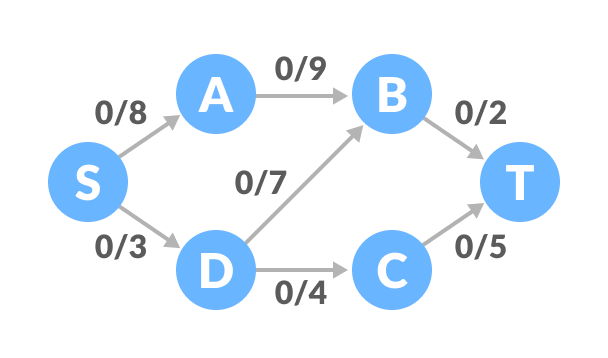Flow network graph example
1. Select any arbitrary path from S to T. In this step, we have selected path S-A-B-T.Find a path
The minimum capacity among the three edges is 2 (B-T). Based on this, update the flow/capacity for each path.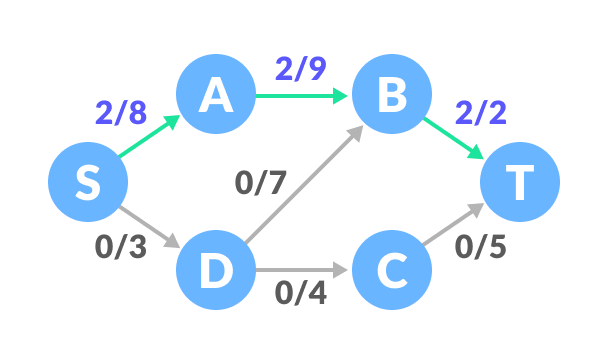Update the capacities
2. Select another path S-D-C-T. The minimum capacity among these edges is 3 (S-D).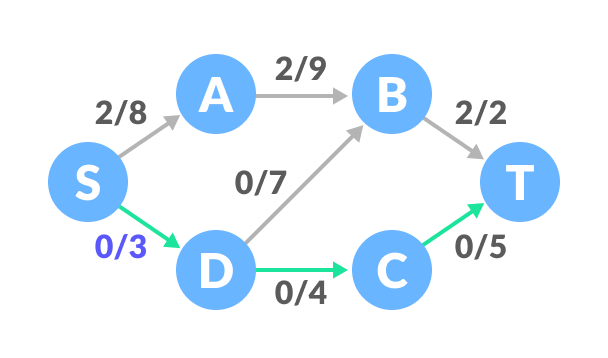Find next path
Update the capacities according to this.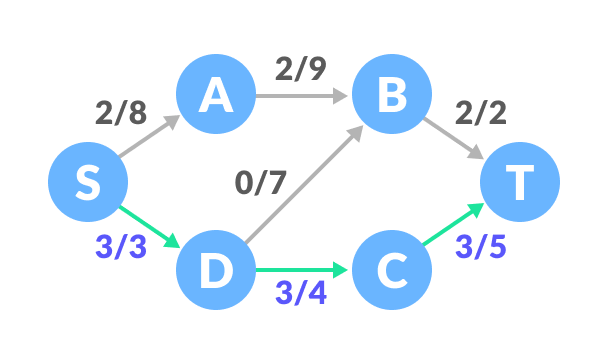Update the capacities
3. Now, let us consider the reverse-path B-D as well. Selecting path S-A-B-D-C-T. The minimum residual capacity among the edges is 1 (D-C).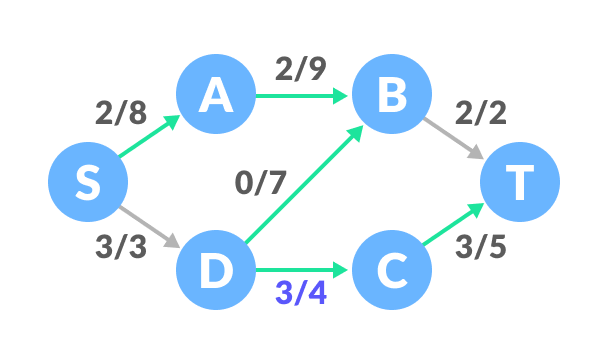Find next path
Updating the capacities.Update the capacities
The capacity for forward and reverse paths are considered separately.
4. Adding all the flows = 2 + 3 + 1 = 6, which is the maximum possible flow on the flow network.

Note that if the capacity for any edge is full, then that path cannot be used.

## Python, Java and C/C++ Examples

``````# Ford-Fulkerson algorith in Python

from collections import defaultdict

class Graph:

def __init__(self, graph):
self.graph = graph
self. ROW = len(graph)

# Using BFS as a searching algorithm
def searching_algo_BFS(self, s, t, parent):

visited = [False] * (self.ROW)
queue = []

queue.append(s)
visited[s] = True

while queue:

u = queue.pop(0)

for ind, val in enumerate(self.graph[u]):
if visited[ind] == False and val > 0:
queue.append(ind)
visited[ind] = True
parent[ind] = u

return True if visited[t] else False

# Applying fordfulkerson algorithm
def ford_fulkerson(self, source, sink):
parent = [-1] * (self.ROW)
max_flow = 0

while self.searching_algo_BFS(source, sink, parent):

path_flow = float("Inf")
s = sink
while(s != source):
path_flow = min(path_flow, self.graph[parent[s]][s])
s = parent[s]

# Adding the path flows
max_flow += path_flow

# Updating the residual values of edges
v = sink
while(v != source):
u = parent[v]
self.graph[u][v] -= path_flow
self.graph[v][u] += path_flow
v = parent[v]

return max_flow

graph = [[0, 8, 0, 0, 3, 0],
[0, 0, 9, 0, 0, 0],
[0, 0, 0, 0, 7, 2],
[0, 0, 0, 0, 0, 5],
[0, 0, 7, 4, 0, 0],
[0, 0, 0, 0, 0, 0]]

g = Graph(graph)

source = 0
sink = 5

print("Max Flow: %d " % g.ford_fulkerson(source, sink))``````
``````// Ford-Fulkerson algorith in Java

import java.util.LinkedList;

class FordFulkerson {
static final int V = 6;

// Using BFS as a searching algorithm
boolean bfs(int Graph[][], int s, int t, int p[]) {
boolean visited[] = new boolean[V];
for (int i = 0; i < V; ++i)
visited[i] = false;

LinkedList<Integer> queue = new LinkedList<Integer>();
queue.add(s);
visited[s] = true;
p[s] = -1;

while (queue.size() != 0) {
int u = queue.poll();

for (int v = 0; v < V; v++) {
if (visited[v] == false && Graph[u][v] > 0) {
queue.add(v);
p[v] = u;
visited[v] = true;
}
}
}

return (visited[t] == true);
}

// Applying fordfulkerson algorithm
int fordFulkerson(int graph[][], int s, int t) {
int u, v;
int Graph[][] = new int[V][V];

for (u = 0; u < V; u++)
for (v = 0; v < V; v++)
Graph[u][v] = graph[u][v];

int p[] = new int[V];

int max_flow = 0;

# Updating the residual calues of edges
while (bfs(Graph, s, t, p)) {
int path_flow = Integer.MAX_VALUE;
for (v = t; v != s; v = p[v]) {
u = p[v];
path_flow = Math.min(path_flow, Graph[u][v]);
}

for (v = t; v != s; v = p[v]) {
u = p[v];
Graph[u][v] -= path_flow;
Graph[v][u] += path_flow;
}

// Adding the path flows
max_flow += path_flow;
}

return max_flow;
}

public static void main(String[] args) throws java.lang.Exception {
int graph[][] = new int[][] { { 0, 8, 0, 0, 3, 0 }, { 0, 0, 9, 0, 0, 0 }, { 0, 0, 0, 0, 7, 2 },
{ 0, 0, 0, 0, 0, 5 }, { 0, 0, 7, 4, 0, 0 }, { 0, 0, 0, 0, 0, 0 } };
FordFulkerson m = new FordFulkerson();

System.out.println("Max Flow: " + m.fordFulkerson(graph, 0, 5));

}
}``````
``````/ Ford - Fulkerson algorith in C

#include <stdio.h>

#define A 0
#define B 1
#define C 2
#define MAX_NODES 1000
#define O 1000000000

int n;
int e;
int capacity[MAX_NODES][MAX_NODES];
int flow[MAX_NODES][MAX_NODES];
int color[MAX_NODES];
int pred[MAX_NODES];

int min(int x, int y) {
return x < y ? x : y;
}

int head, tail;
int q[MAX_NODES + 2];

void enqueue(int x) {
q[tail] = x;
tail++;
color[x] = B;
}

int dequeue() {
int x = q[head];
head++;
color[x] = C;
return x;
}

// Using BFS as a searching algorithm
int bfs(int start, int target) {
int u, v;
for (u = 0; u < n; u++) {
color[u] = A;
}
head = tail = 0;
enqueue(start);
pred[start] = -1;
while (head != tail) {
u = dequeue();
for (v = 0; v < n; v++) {
if (color[v] == A && capacity[u][v] - flow[u][v] > 0) {
enqueue(v);
pred[v] = u;
}
}
}
return color[target] == C;
}

// Applying fordfulkerson algorithm
int fordFulkerson(int source, int sink) {
int i, j, u;
int max_flow = 0;
for (i = 0; i < n; i++) {
for (j = 0; j < n; j++) {
flow[i][j] = 0;
}
}

// Updating the residual values of edges
while (bfs(source, sink)) {
int increment = O;
for (u = n - 1; pred[u] >= 0; u = pred[u]) {
increment = min(increment, capacity[pred[u]][u] - flow[pred[u]][u]);
}
for (u = n - 1; pred[u] >= 0; u = pred[u]) {
flow[pred[u]][u] += increment;
flow[u][pred[u]] -= increment;
}
// Adding the path flows
max_flow += increment;
}
return max_flow;
}

int main() {
for (int i = 0; i < n; i++) {
for (int j = 0; j < n; j++) {
capacity[i][j] = 0;
}
}
n = 6;
e = 7;

capacity = 8;
capacity = 3;
capacity = 9;
capacity = 7;
capacity = 2;
capacity = 5;
capacity = 7;
capacity = 4;

int s = 0, t = 5;
printf("Max Flow: %d\n", fordFulkerson(s, t));
}``````
``````// Ford-Fulkerson algorith in C++

#include <limits.h>
#include <string.h>

#include <iostream>
#include <queue>
using namespace std;

#define V 6

// Using BFS as a searching algorithm
bool bfs(int rGraph[V][V], int s, int t, int parent[]) {
bool visited[V];
memset(visited, 0, sizeof(visited));

queue<int> q;
q.push(s);
visited[s] = true;
parent[s] = -1;

while (!q.empty()) {
int u = q.front();
q.pop();

for (int v = 0; v < V; v++) {
if (visited[v] == false && rGraph[u][v] > 0) {
q.push(v);
parent[v] = u;
visited[v] = true;
}
}
}

return (visited[t] == true);
}

// Applying fordfulkerson algorithm
int fordFulkerson(int graph[V][V], int s, int t) {
int u, v;

int rGraph[V][V];
for (u = 0; u < V; u++)
for (v = 0; v < V; v++)
rGraph[u][v] = graph[u][v];

int parent[V];
int max_flow = 0;

// Updating the residual values of edges
while (bfs(rGraph, s, t, parent)) {
int path_flow = INT_MAX;
for (v = t; v != s; v = parent[v]) {
u = parent[v];
path_flow = min(path_flow, rGraph[u][v]);
}

for (v = t; v != s; v = parent[v]) {
u = parent[v];
rGraph[u][v] -= path_flow;
rGraph[v][u] += path_flow;
}

// Adding the path flows
max_flow += path_flow;
}

return max_flow;
}

int main() {
int graph[V][V] = {{0, 8, 0, 0, 3, 0},
{0, 0, 9, 0, 0, 0},
{0, 0, 0, 0, 7, 2},
{0, 0, 0, 0, 0, 5},
{0, 0, 7, 4, 0, 0},
{0, 0, 0, 0, 0, 0}};

cout << "Max Flow: " << fordFulkerson(graph, 0, 5) << endl;
}``````

## Ford-Fulkerson Applications

• Water distribution pipeline
• Bipartite matching problem
• Circulation with demands